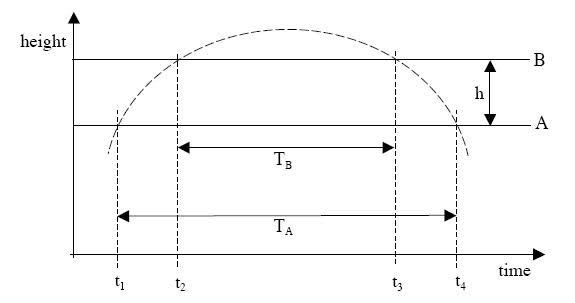## Homework Statement

The acceleration due to gravity can be measured by firing a projectile upward and
measuring the time it takes to pass two given points in both directions. The figure below
is a plot of height versus time and shows that the time the projectile takes to pass a
horizontal line A in both directions is TA, and the time it takes to pass a horizontal line B
in both directions is TB. Derive an expression for g, the acceleration due to gravity. Your
expression should involve only h, TA, and TB. State any assumptions you make.## The Attempt at a Solution

mjsd
Homework Helper
this is a constant acceleration problem.
need standard kinematics equations for constant accelerations
relate relevant variables based on the plot (ie. what you know) and then solve those resultant simultaneous equations

this is a constant acceleration problem.
need standard kinematics equations for constant accelerations
relate relevant variables based on the plot (ie. what you know) and then solve those resultant simultaneous equations

Could you guide me through them!
I tried but i couldn't make it to work.

mjsd
Homework Helper
first, learn the equations (if not the derivation of them as well)

http://www.ac.wwu.edu/~vawter/PhysicsNet/Topics/Kinematics/ConstantAccEqMotion.html [Broken]

and understand how to interpret graphs

http://www.ac.wwu.edu/~vawter/PhysicsNet/Topics/Kinematics/SpaceTime.html [Broken]

Last edited by a moderator:

I'm working this problem right now. Where do you start? I mean do you go from when it passes A the first time to when it passes A the second time, similiarly with B? Do you go from ground to A, to A to B, to B to B again on the way down, to B to the ground?

If you measure from A to A and B to B I get:

0=(V1)*(Ta)-.5*g*(Ta)^2
and
0=(V2)*(Tb)-.5*g*(Tb)^2

But how are you supposed to related them? And How do you get rid of the V's?

If I do it the second Way I get times that arn't right like (t1-t2).What are you defining V1 and V2 as?

I'm Defining V1 as the velocity of the object as it passes the A line, and V2 as the velocity as the object passes the B line. Is this correct?

Ok, can someone at least tell me how they would start this freaking problem?

rl.bhat
Homework Helper

If u is the velocity of projection, at t1 the velocity will be
vA = u - g*t1. ........(1)
At t4 the velocity will be
v2 = -vA = u - g*t4....(2). From equ. 1 and 2 we get
2vA = g*(t2 - t1 ) or vA = g/2*(t4 - t1) .....(3)
Similarly vB = g/2*( t3 - t2).....(4)
Now use the vB^2 - vA^2 = 2g*h find g.